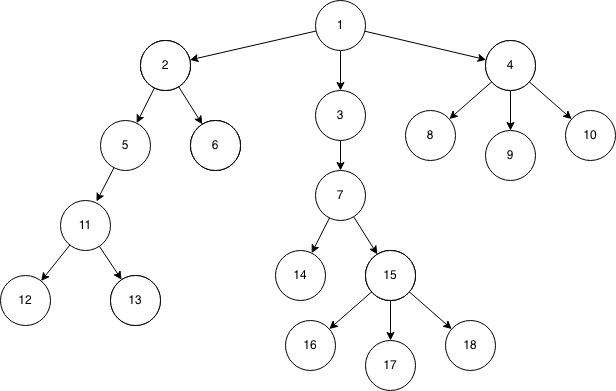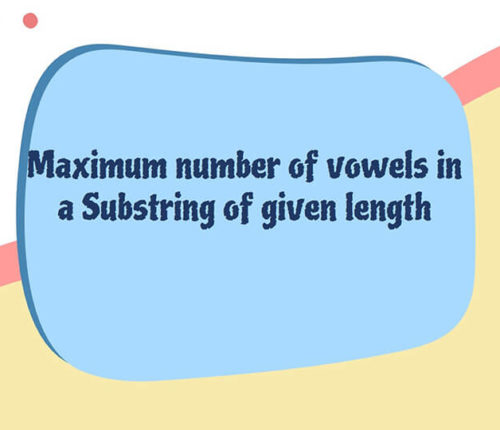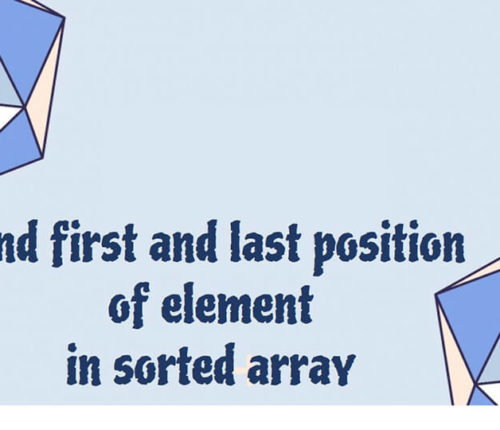# Lowest Common Ancestor (LCA) for n-ary Tree

If you want to practice data structure and algorithm programs, you can go through Java coding interview questions.

In this post, we will see about Lowest Common Ancestor for n-ary Tree.

## Problem

Given a n-ary tree also known as a Generic Tree and also two nodes. You need to find the Lowest common ancestor of the two given nodes.
A n-ary tree is basically a tree with any number of children.
Lowest Common Ancestor is the node which is the common ancestor for both the nodes which is closest to them or we can say, farthest from root.

Consider the Given Tree,Input Format:
In the first line, given n, the number of nodes, following that n-1 lines contains two integers, the first one representing parent, second one is the child.
After that, given two Integers, whose LCA is to be found.

For Eg:-

Input :
18
1 2
1 3
1 4
2 5
2 6
3 7
4 8
4 9
4 10
5 11
11 12
11 13
7 14
7 15
15 16
15 17
15 18
6 13
Output : 2

## Solution

Approach – I :

In this approach, we will basically use the find node algorithm, we start with root node, keep searching for both of the given nodes whose LCA id to be found say(u, v).

• There are two cases When a node will be LCA :
• (i) When itself is one of the node i.e equal to u or v, and other node falls in the subtree of its children.
• (ii) When both the nodes falls in the DIFFERENT subtrees of its children, if they fall in the
same subtree of its child, then this node might not be the LCA.
• So we start from root node, keep on recurring in preorder, searching for u and v. If we find any node of u and v then we return true, as this might be the LCA, even though we have a different condition for considering it as the LCA which we will see later.
• When we recur for children of any node, we keep a counter of how many child are giving a true as result, because :
• (i) If exactly Two of its children return true, this means that the current node is the LCA (As discussed in the second case).
• (ii) If one of its child return true and it itself is one of the node whose LCA to be found then this node is LCA (As discussed in the First case).

As we are setting LCA in postorder, we need to make sure that we set it only once.
As we are recurring in preorder, visiting every node, so the time complexity of this approach would be O(n).

Approach – II :

This approach also solves the give problem in linear time i.e with the worst time complexity of O(n).
We basically use Root to node path to find out the LCA of two nodes.

• Lets first discuss how we find root to node path :
1. We keep on traversing the tree in preorder basically looking for the node whose path we are currently finding.
2. Once we find the node, there is no need for further calls for siblings or for child, we will return true and for stopping those further calls, we check if we already got a true already, if yes, then this means that we already found the node.
3. We add the node into our path and while backtracking in postorder, we add only one node at a level, because if we keep adding the nodes in postorder without any check, then it is more likely that we will also add the sibling for a node whose root to node path we are actually finding.
4. Now after we got our root to node path, we will traverse both the paths starting from extreme right end for both, and keep moving until we get a different node on both pointers, this means that the node which we are currently at is the node at which the path for both given nodes(whose LCA is to be found) are diverged and hence the previous node is the LCA of both nodes.

}

When you run above program, you will get below output:

18
1 2
1 3
1 4
2 5
2 6
3 7
4 8
4 9
4 10
5 11
11 12
11 13
7 14
7 15
15 16
15 17
15 18
6 13
[6, 2, 1] [13, 11, 5, 2, 1] Output: 2

That’s all about Lowest Common Ancestor for any K-ary Tree

import_contacts

### You may also like:## Related Posts

•18 June

### Maximum Number of Vowels in a Substring of Given Length

Table of ContentsApproach – 1 Generate All Substrings Using substring() MethodApproach – 2 Using Sliding Window Method (Linear Time Solution) In this article, we will look at an interesting problem related to the Strings and [Sliding-Window Algorithm](https://java2blog.com/sliding-window-maximum-java/ “Sliding-Window Algorithm”). The problem is : "Given a String we have to Find the Maximum Number of Vowel […]

•04 June

### Search for a range Leetcode – Find first and last position of element in sorted array

Table of ContentsApproach 1 (Using Linear Search)Approach 2 (Using Modified Binary Search-Optimal) In this article, we will look into an interesting problem asked in Coding Interviews related to Searching Algorithms. The problem is: Given a Sorted Array, we need to find the first and last position of an element in Sorted array. This problem is […]

•30 April

### Convert Postfix to Infix in Java

Learn about how to convert Postfix to Infix in java.

•30 April

### Convert Prefix to Postfix in Java

Learn about how to convert Prefix to Postfix in java.

•16 April

### Data Structures in java

•